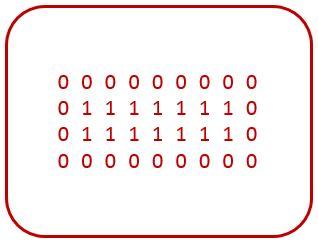# C program to print binary rectangular pattern where all boundary elements are 0 and all inner elements are 1

• Write a program in C to print binary rectangle pattern as given below.
• How to draw a rectangle pattern in C where all boundary elements are 0.

### BINARY RECTANGLE#### Required Knowledge

Algorithm to print rectangular pattern of binary digit 0 and 1
This program is similar to rectangle star pattern. At every position of rectangle we will check for boundary condition (first and last row and column of rectangle). If true, then we print 0 othewise 1.

## C program to print binary rectangle pattern

```#include<stdio.h>

int main(){
int rows, cols , i, j;

printf("Enter rows and columns of rectangle\n");
scanf("%d %d", &rows, &cols);

/* Row iterator for loop */
for(i = 0; i < rows; i++){
/* Column iterator for loop */
for(j = 0; j < cols; j++){
/* Check if currely position is a boundary position */
if(i==0 || i==rows-1 || j==0 || j==cols-1)
printf("0");
else
printf("1");
}
printf("\n");
}
return 0;
}
```
Output
```Enter rows and columns of rectangle
4 7
0 0 0 0 0 0 0
0 1 1 1 1 1 0
0 1 1 1 1 1 0
0 0 0 0 0 0 0
```

Related Topics
 C program hollow rectangle pattern C program rectangle star pattern C program hollow square star pattern C program binary triangle pattern C program heart shape star pattern C program same row element triangle pattern C program prime number triangle pattern C program palindrome triangle pattern C program diamond star pattern List of all C pattern programs